# Suntsu Electronics Launches Easy-to Use Calculators!Introducing Suntsu’s passive device technical references!

Our team has been working hard to create easy-to-use calculators and converters for 6 of the most common passive device technical references.

## Capacitance Calculator

Capacitance is an object’s ability to store electric charges. It relies on the shape of the capacitor and the choice of the dielectric material used in between the plates. Two types of calculations can be done with capacitance: series or parallel, depending on the arrangement in the circuit.

## Capacitance Converter

Suntsu’s capacitance converter will help you convert between four units (F, pF, nF, µF). To avoid confusion, when an electronic component or diagram mentions a value in a different metric than what you need, our calculator is here to assist you.

## Inductance Converter

Inductance is measured with an SI unit known as the henry (H). It is defined as the ratio between the induced voltage and the rate of change of current causing it. One henry (H) is equivalent to one volt divided by one ampere per second. If a current changing at the rate of one ampere per second induces an electromotive force of one volt, the circuit has an inductance of one henry (H).

## Ohm’s Law Calculator

Ohm’s law explains the relationship between voltage, current, and resistance in an electrical circuit. Now considered one of the basic laws of physics, it states that the current through a conductor between two points is directly proportional to the potential difference across the two points.

## PPM to % Converter

PPM, parts per million, is a way of referring to very small concentrations of substances, usually in water or soil. This makes it a popular calculation for agricultural, water treatment industries, and chemists. The basic formula for PPM begins by dividing the weight or number of defects by volume and then multiplying the result by 1,000,000.

## Resistor Color Code

The values of resistors are indicated with color codes, or in this case, color bands. There is a recognized international standard for this coding. Most resistors have anywhere between three to six bands. Two bands indicate the resistance value, and one band serves as a multiplier.

Need more help or have other converters and calculators you want us to create? Connect with us!

## Get A Quote

Please use the form below to create a request for a quote.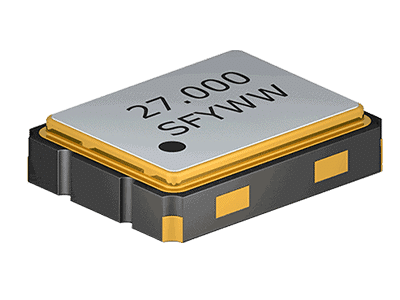Frequency Control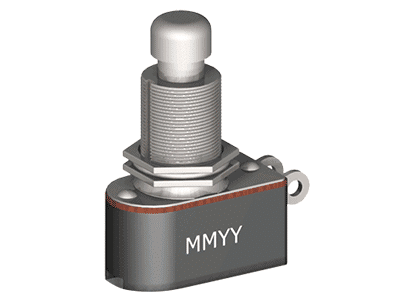Switches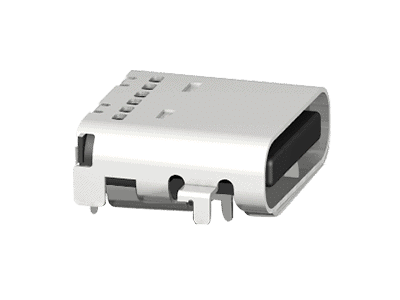Interconnect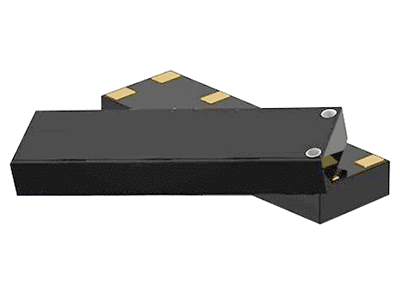Antennas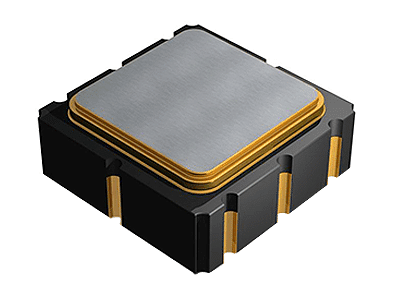Saw Filters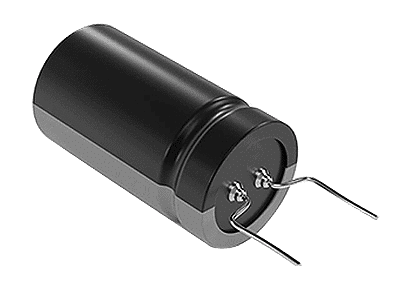Franchised Products

We help our customers build better products, save time, save money, and improve cash-flow/lead-times through inventory management programs.Sourcing/
ManufacturingEngineering
ServicesInventory
Management

Helping Tech Companies Create What’s Next!